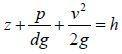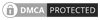## Bernoullis Theorem

Bernoulli's theorem is a method of expressing the law of conservation of energy to the flow of fluids.Bernoulli's principle stats that, in the flow of fluid (a liquid or gas), an increase in velocity occurs simultaneously with decrease in pressure. That statement is a simplification of Bernoulli's equation (below) which plots the situation at any point on a streamline of the fluid flow and applies the law of conservation of energy to flow. Put another way, the total energy of the flow at any point along a horizontal pipe is equal to the sum of the pressure head, the velocity head and the elevation in the absence of friction. This is a principle of considerable importance to those concerned with flow in sprinkler pipeworkWhen

z = Potential head or elevation
p = Pressure
v = Velocity
g = Acceleration of gravity
d = Density of fluid

If friction losses are ignored and no energy is added or removed from the pipe the total head h, in the above equation will be constant for any point in the fluid. However in practice energy will increase and decrease with the effect of pumps and friction loss and this must be included in the Bernoulli's equation. All practical formulas for the flow of fluids are derived from the Bernoulli's theorem with modifications to account for losses due to friction.Canute LLP
15 Queen Square
Leeds, West Yorkshire
United Kingdom LS2 8AJ

t: +44 (0) 113 328 0350
e: info@canutesoft.com

 Copyright © 2021, Canute LLPRegistered in England & Wales, Partnership No. OC305985